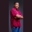Related Tags

scala
swap
communitycreator

# How to swap two numbers in ScalaTheodore Kelechukwu Onyejiaku

### Overview

Swapping of variables is when we assign the value of one variable to another programmatically. In this shot, we'll see how we can swap the value of one variable with another, since there is no default method that can allow us to do this.

There are basically two ways to achieve this:

• With a third variable
• Without a third variable

Let's see these possibilities in the syntax below.

### Syntax

c = a;
a = b;
b = c;
Syntax to swap two variables using third variable
a = a + b;
b = a - b;
a = a - b;
Syntax to swap two number variables without a third variable

### Parameters

c: This is the third variable.

b: This is the second number variable.

a: This is the first number variable.

### Examples

We'll see swapping with and without using a third variable.

#### With third variable

object Main extends App {
var a = 5
var b = 10

// print values before swapping
println("Before Swapping a = " +a+ " b =" +b)

// swap
var c = a
a = b
b = c

// print the values after swapping
println("After Swapping a = " +a+ " b =" +b)
}
Swap variable values with a third variable

#### Explanation

• Lines 2–3: We create our two number variables and initialize them.
• Line 6: We print the values before swapping.
• Line 9–11: We swap the variable values using a third variable, c.
• Line 14: We finally print the swapped values.

#### Without third variable

object Main extends App {
var a = 5
var b = 10

// print values before swapping
println("Before Swapping a = " +a+ " b =" +b)

// swap
a = a + b
b = a - b
a = a - b

// print the values after swapping
println("After Swapping a = " +a+ " b =" +b)
}
Swap number values without a third variable in Scala

#### Explanation

• Line 2–3: We create our two number variables and initialize them.
• Line 6: We print the values before swapping.
• Line 9–11: We swap the variable values without using any third variable.
• Line 14: We finally print the swapped values.

RELATED TAGS

scala
swap
communitycreator

CONTRIBUTORTheodore Kelechukwu Onyejiaku
RELATED COURSES

View all Courses

Keep Exploring

Learn in-demand tech skills in half the time